Next: White-Dwarf Stars Up: Three-Dimensional Quantum Mechanics Previous: Particle in a Box

# Degenerate Electron Gases

Considerelectrons trapped in a cubic box of dimension. Let us treat the electrons as essentially non-interacting particles. According to Sect. 6.3, the total energy of a system consisting of many non-interacting particles is simply the sum of the single-particle energies of the individual particles. Furthermore, electrons are subject to the Pauli exclusion principle (see Sect. 6.5), since they are indistinguishable fermions. The exclusion principle states that no two electrons in our system can occupy the same single-particle energy level. Now, from the previous section, the single-particle energy levels for a particle in a box are characterized by the three quantum numbers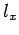,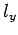, and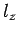. Thus, we conclude that no two electrons in our system can have the same set of values of,, and. It turns out that this is not quite true, because electrons possess an intrinsic angular momentum called spin (see Cha. 10). The spin states of an electron are governed by an additional quantum number, which can take one of two different values. Hence, when spin is taken into account, we conclude that a maximum of two electrons (with different spin quantum numbers) can occupy a single-particle energy level corresponding to a particular set of values of,, and. Note, from Eqs. (504) and (505), that the associated particle energy is proportional to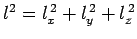.

Suppose that our electrons are cold: i.e., they have comparatively little thermal energy. In this case, we would expect them to fill the lowest single-particle energy levels available to them. We can imagine the single-particle energy levels as existing in a sort of three-dimensional quantum number space whose Cartesian coordinates are,, and. Thus, the energy levels are uniformly distributed in this space on a cubic lattice. Moreover, the distance between nearest neighbour energy levels is unity. This implies that the number of energy levels per unit volume is also unity. Finally, the energy of a given energy level is proportional to its distance,, from the origin.

Since we expect cold electrons to occupy the lowest energy levels available to them, but only two electrons can occupy a given energy level, it follows that if the number of electrons,, is very large then the filled energy levels will be approximately distributed in a sphere centered on the origin of quantum number space. The number of energy levels contained in a sphere of radius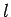is approximately equal to the volume of the sphere--since the number of energy levels per unit volume is unity. It turns out that this is not quite correct, because we have forgotten that the quantum numbers,, andcan only take positive values. Hence, the filled energy levels actually only occupy one octant of a sphere. The radiusof the octant of filled energy levels in quantum number space can be calculated by equating the number of energy levels it contains to the number of electrons,. Thus, we can write(506)

Here, the factor 2 is to take into account the two spin states of an electron, and the factor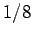is to take account of the fact that,, andcan only take positive values. Thus,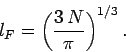(507)

According to Eq. (504), the energy of the most energetic electrons--which is known as the Fermi energy--is given by(508)

whereis the electron mass. This can also be written as(509)

whereis the number of electrons per unit volume (in real space). Note that the Fermi energy only depends on the number density of the confined electrons.

The mean energy of the electrons is given by(510)

since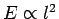, and the energy levels are uniformly distributed in quantum number space inside an octant of radius. Now, according to classical physics, the mean thermal energy of the electrons is, where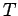is the electron temperature, andthe Boltzmann constant. Thus, if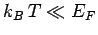then our original assumption that the electrons are cold is valid. Note that, in this case, the electron energy is much larger than that predicted by classical physics--electrons in this state are termed degenerate. On the other hand, ifthen the electrons are hot, and are essentially governed by classical physics--electrons in this state are termed non-degenerate.

The total energy of a degenerate electron gas is(511)

Hence, the gas pressure takes the form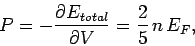(512)

since[see Eq. (508)]. Now, the pressure predicted by classical physics is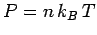. Thus, a degenerate electron gas has a much higher pressure than that which would be predicted by classical physics. This is an entirely quantum mechanical effect, and is due to the fact that identical fermions cannot get significantly closer together than a de Broglie wavelength without violating the Pauli exclusion principle. Note that, according to Eq. (509), the mean spacing between degenerate electrons is(513)

where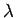is the de Broglie wavelength. Thus, an electron gas is non-degenerate when the mean spacing between the electrons is much greater than the de Broglie wavelength, and becomes degenerate as the mean spacing approaches the de Broglie wavelength.

In turns out that the conduction (i.e., free) electrons inside metals are highly degenerate (since the number of electrons per unit volume is very large, and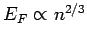). Indeed, most metals are hard to compress as a direct consequence of the high degeneracy pressure of their conduction electrons. To be more exact, resistance to compression is usually measured in terms of a quantity known as the bulk modulus, which is defined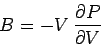(514)

Now, for a fixed number of electrons,[see Eqs. (508) and (512)]. Hence,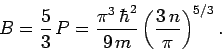(515)

For example, the number density of free electrons in magnesium is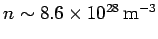. This leads to the following estimate for the bulk modulus: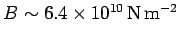. The actual bulk modulus is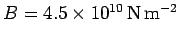.Next: White-Dwarf Stars Up: Three-Dimensional Quantum Mechanics Previous: Particle in a Box
Richard Fitzpatrick 2010-07-20